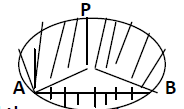mycollegehive
Find the area of the major segment APB, of a circle of radius 35 cm and ∟AOB = 90˚ (π = 22/7)
CBSE class-10 math areas-related-to-circles0Find the area of the major segment APB, of a circle of radius 35 cm and ∟AOB = 90˚ (π = 22/7)

\20 viewsShareFollowSchool not set Nigeria
01 August 2020University of Benin Nigeria
01 August 20200∟AOB = 90˚, thus, AOB is a right angle.

Area of major segment APB = Area of major sector APB + Area of triangle AOB

Area of major sector APB = 1/2 (length of arc x radius)

length of arc = θ/360 x 2πr

Area of major sector APB = 1/2 (θ/360 x 2πr x r)

= 1/2 (θ/360 x 2πr2)

= θ/360 πr2

Area of triangle AOB = 1/2 base x height

base = radius of the circle

height = radius of the circle

Thus, area of triangle AOB = 1/2 r x r

= 1/2 r2

Therefore, the area of the major segment APB = θ/360 πr+ 1/2 r2

where r is the radius, θ is the center angle

r = 35cm

for the major arc, θ = 360 - 90 = 270

Area of major segment APB = (270/360 x π(35)2) + (1/2 (35)2)

= (3675π/4) + (1225/2)

but π = 22/7,

Hence, area the major segment APB = (3675/4 x 22/7) + (1225/2)

= 2887.5 + 612.5

= 3500 cm2Share### Related Tags

CBSE

0 followers

56 questionsclass-10

0 followers

56 questionsmath

2 followers

985 questions